## Center of gravity; and Rotational variables

10-25-99

Sections 7.8 - 8.3

### Center of gravity

The center of gravity of an object is the point you can suspend the object from without there being any rotation because of the force of gravity, no matter how the object is oriented. If you suspend an object from any point, let it go and allow it to come to rest, the center of gravity will lie along a vertical line that passes through the point of suspension. Unless you've been exceedingly careful in balancing the object, the center of gravity will generally lie below the suspension point.

The center of gravity is an important point to know, because when you're solving problems involving large objects, or unusually-shaped objects, the weight can be considered to act at the center of gravity. In other words, for many purposes you can assume that object is a point with all its weight concentrated at one point, the center of gravity.

For any object, the x-position of the center of gravity can be found by considering the weights and x-positions of all the pieces making up the object:A similar equation would allow you to find the y position of the center of gravity.

The center of mass of an object is generally the same as its center of gravity. Very large objects, large enough that the acceleration due to gravity varies in different parts of the object, are the only ones where the center of mass and center of gravity are in different places.

### Neat facts about the center of gravity

Fact 1 - An object thrown through the air may spin and rotate, but its center of gravity will follow a smooth parabolic path, just like a ball.

Fact 2 - If you tilt an object, it will fall over only when the center of gravity lies outside the supporting base of the object.

Fact 3 - If you suspend an object so that its center of gravity lies below the point of suspension, it will be stable. It may oscillate, but it won't fall over.

### Rotational variables

We'll now switch the focus from straight-line motion to rotational motion. If you can do one-dimensional motion problems, which involve straight-line motion, then you should be able to do rotational motion problems, because a circle is just a straight line rolled up. To solve rotational kinematics problems, a set of four equations is used; these are essentially the one-dimensional motion equations in disguise.

If you spin a wheel, and look at how fast a point on the wheel is spinning, the answer depends on how far away the point is from the center. Velocity, then, isn't the most convenient thing to use when you're dealing with rotation, and for the same reason neither is displacement, or acceleration; it is often more convenient to use their rotational equivalents. The equivalent variables for rotation are angular displacement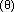(angle, for short); angular velocity, and angular acceleration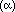. All the angular variables are related to the straight-line variables by a factor of r, the distance from the center of rotation to the point you're interested in.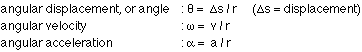Although points at different distances from the center of a rotating wheel have different velocities, they all have the same angular velocity, so they all go around the same number of revolutions per minute, and the same number of radians per second. Angles (angular displacements, that is) are generally measured in radians, which is the most convenient unit to work with. A radian is an odd unit in physics, however, because it is treated as being unitless, and is often put in or taken out whenever it's convenient to do so.

It is helpful to recognize the parallel between straight-line motion and rotational motion. Writing down the four rotational kinematics equations reinforces that. Any equation dealing with rotation can be found from its straight-line motion equivalent by substituting the corresponding rotational variables.

The straight-line motion kinematics equations apply for constant acceleration, so it follows that the rotational kinematics equations apply when the angular acceleration is constant. The equations should look familiar to you:The equations are the same as the constant-acceleration equations for 1-D motion, substituting the rotational equivalents of the straight-line motion variables.

### A rotational example

Consider an example of a spinning object to see how the rotational kinematics equations are applied. Imagine a ferris wheel that is rotating at the rate of 1 revolution every 8 seconds. The operator of the wheel decides to bring it to a stop, and puts on the brake; the brake produces a constant deceleration of 0.11 radians/s2.

(a) If your seat on the ferris wheel is 4.2 m from the center of the wheel, what is your speed when the wheel is turning at a constant rate, before the brake is applied?
(b) How long does it take before the ferris wheel comes to a stop?
(c) How many revolutions does the wheel make while it is coming to a stop?
(d) How far do you travel while the wheel is slowing down?

(a) The wheel is rotating at a rate of 1 revolution every 8 seconds, or 0.125 rev/s. This is the initial angular velocity. It is often most convenient to work with angular velocity in units of radians/s; doing the conversion gives:Your speed is simply this angular velocity multiplied by your distance from the center of the wheel: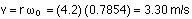(b) We've calculated the initial angular velocity, the final angular velocity is zero, and the angular acceleration is -0.11 rad/s2. This allows the stopping time to be found:(c) To find the number of revolutions the wheel undergoes in this 7.14 seconds, one way to do it is to use the equation: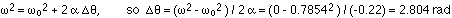This can be converted to revolutions: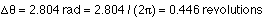(d) To figure out the distance you traveled while the wheel was slowing down. the angular displacement (in radians) can be converted to a displacement by multiplying by r: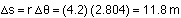### Tangential velocity; tangential acceleration

In uniform circular motion (motion in a circle at a constant speed), there is always a net acceleration (the centripetal acceleration) towards the center of the circular path. In non-uniform circular motion the speed is not constant, and there are two accelerations, the centripetal acceleration towards the center of the circle, and the tangential acceleration. The tangential acceleration is tangent to the circle, pointing in the direction the object is traveling if the object is speeding up, and the opposite way if the object is slowing down.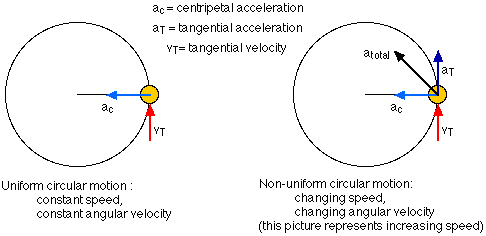The two vector diagrams show an object undergoing uniform circular motion (constant angular velocity), and an object experiencing non-uniform circular motion (varying angular velocity). For uniform circular motion, the centripetal acceleration points towards the center of the circle, and the velocity points in the direction the object is traveling. This is tangent to the circular path, so we call it the tangential velocity. For non-uniform circular motion, the centripetal acceleration and tangential velocity are still there, and there is also a tangential acceleration in the direction the object is traveling. The net acceleration is the vector sum of the centripetal and tangential accelerations. Just as we separate everything out into x and y components when analyzing a projectile motion question, we can always separate things out into the tangential and radial (towards the center) directions in non-uniform circular motion.

Note that the centripetal acceleration is connected to the tangential velocity, through the usual v2 / r relationship, while the tangential acceleration is connected to any change in the tangential speed.

### Rolling

When an object such as a wheel or a ball rolls, it does not slip where it makes contact with the ground. With a car or a bicycle tire, there is friction between the tire and the road, and if the tire is rolling then the frictional force is a static force of friction. This is because there is no slipping, so the point on the tire in contact with the road is instantaneously at rest.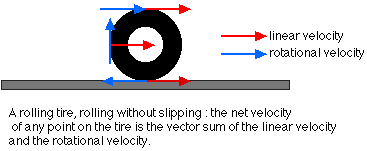This is somewhat counter-intuitive, but it comes about because the velocity of each point on the tire is a sum of the linear velocity associated with the car (or bike) moving, and the rotational velocity associated with the tire rolling. For a point on the outside of the tire, the rotational speed happens to be equal to the linear speed of the car: this is because each time the tire makes a complete revolution, the car will have traveled a distance equal to the circumference of the tire, so the linear distance and the rotational distance are the same for the same time interval. For a point on the top of a tire, the two velocities are in the same direction, so the total velocity at the top of a tire is twice the linear velocity of the car; for a point at the bottom of a tire, the two velocities are in opposite directions, so the total velocity is zero there.

Consider a bike on a flat road. You climb on, and start pedaling, and the bike accelerates forward, with both tires rolling along the road. As the bike accelerates, which way does friction act? The answer depends on which tire you consider. Think about what would happen if there was no friction between the tires and the road. When you pedal, the chain causes the rear wheel to spin. With no friction, the rear tire would spin on the road and the bike wouldn't move. Friction opposes this tendency, so it points in the direction you're accelerating on the bike; it's static friction, because the tire does not slip, it rolls.

The front tire, on the other hand, is not being spun by the chain, so with no friction it wouldn't spin at all. Friction is what makes it spin, then, so it must point opposite to the way the bike is accelerating, and, again, it's static friction because the tire does not slip on the road.

Once you've accelerated the bike and you're going at a constant speed, the frictional forces don't have to be as large. The friction on the rear tire has to provide enough force to overcome the resistance forces (rolling resistance, air resistance, friction in the wheel bearings) tending to slow you and the bike down. The friction on the front tire has to do even less, because all it has to do is keep the front tire spinning at a constant rate.